Next: 3.3 Determination of Capacitances by Quasi Static Approximation Up: 3 Measurement and Parameter Extraction Previous: 3.1 DC and RF Measurements

3.2 Small Signal Equivalent Circuit Parameter Extraction

The HEMT can be described by an equivalent circuit model shown in  Figure 3.1. To extract the model parameters from measured S­parameters a value for each parameter of the model is assumed and S­parameters are calculated based on the equivalent circuit. The chosen values are then optimized by minimizing the differences between the calculated and the measured S­parameters.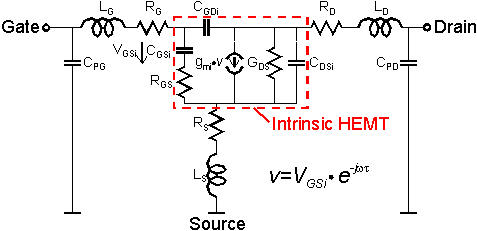Figure 3.1 Equivalent circuit model for parameter extraction. It includes the intrinsic device, the series resitances to all three terminals as well as the parasitic capacitances and inductances of the contacting network.

The transit frequencies fT and fmax can be traced back to equivalent circuit parameters. From the intrinsic device the well known approximation for the current gain cut-off frequency fT can be derived as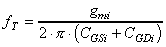. (10)

Extrinsic values of gm and CGS can be calculated by gm = gmi /(1+gmiRs) and CGS = CGSi (1+gmRs). In the active bias region of a HEMT (VDS > 0.5 V and VGS > VT) CGDi is only about 15 % of CGSi. Therefore fT can also be approximated using the extrinsic gm and the extrinsic total gate capacitance CG = CGS + CGDi in (10).

According to [37, 38] fmax can also be approximated with equivalent circuit parameters by: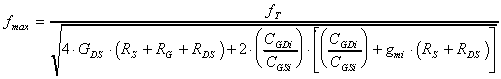. (11)

For measuring purposes the devices are embedded in a network of coplanar lines and contacting pads. These will be not included in the simulation area as described in Chapter 5. The contacting network can be neglected in first order for DC measurements but must be taken into account when simulated and measured RF parameters such as fT and fmax are compared. Thus, the parasitic elements LG, LD, LS, CPG and CPD have to be included in the equivalent circuit model.

Based on the equivalent circuit the parameters can be extracted from measured S­parameters. In the extraction procedure it is difficult to distinguish between the parasitic pad capacitances CPG and CPD and the gate source capacitance CGS because they are connected in parallel and therefore only their sum can be determined reliably. In first order CPG and CPD are the only capacitances which do not scale with the gate width Lw. This can be used to extract these values in a different way.

The measured value of fT includes all capacitances. The total capacitance can be determined rearranging (10) to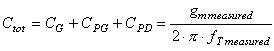. (12)

As gm, CGS and CGD scale linearly with Lw and both CPG and CPD are constant for all the devices the sum CPG + CPD can be determined by extrapolating Ctot to Lw = 0. The calculated Ctot using measured gm and fT for different bias points is shown in  Figure 3.2. The intercept for all linear fits is very close to about 25fF. This value is used for parameter extraction of all measured devices. More details of this deembedding procedure are described in .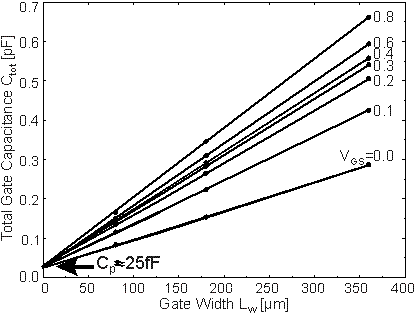Figure 3.2 Total capacitance of HEMTs versus gate width at VDS=2.0V. The capacitances are calculated using (12) with measured gm and fT of devices with gate widths Lw= 80, 180, 360 µm. For all VGS a linear fit can be found. The intercept determines the constant part of Ctot, i. e. CPG + CPG.

The schematic of the extrinsic device used for simulation is shown in  Figure 2.1 It corresponds to the intrinsic device indicated by the dashed box in  Figure 3.1 and the parasitic resistances RS, RG, and RD. The deembedded device parameters will be the basis for comparison between measured and simulated data.

Helmut Brech
1998-03-11